JMSLTM Numerical Library 7.2.0
com.imsl.math

• All Implemented Interfaces:
Serializable

```public class ConjugateGradient
extends Object
implements Serializable```
Solves a real symmetric definite linear system using the conjugate gradient method with optional preconditioning.

Class `ConjugateGradient` solves the symmetric positive or negative definite linear system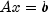using the conjugate gradient method with optional preconditioning. This method is described in detail by Golub and Van Loan (1983, Chapter 10), and in Hageman and Young (1981, Chapter 7).

The preconditioning matrix M is a matrix that approximates A, and for which the linear system Mz=r is easy to solve. These two properties are in conflict; balancing them is a topic of current research. If no preconditioning matrix is specified,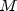is set to the identity, i.e.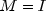.

The number of iterations needed depends on the matrix and the error tolerance. As a rough guide,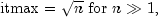where n is the order of matrix A.

See the references for details.

Let M be the preconditioning matrix, let b,p,r,x and z be vectors and letbe the desired relative error. Then the algorithm used is as follows: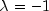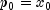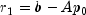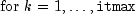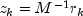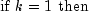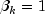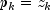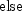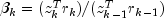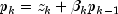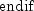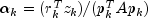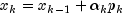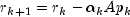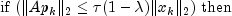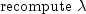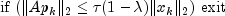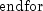Here,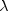is an estimate of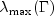, the largest eigenvalue of the iteration matrix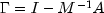. The stopping criterion is based on the result (Hageman and Young 1981, pp. 148-151)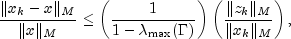where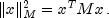It is also known that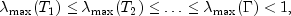where the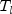are the symmetric, tridiagonal matrices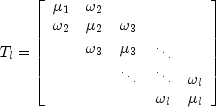with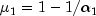and, for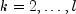,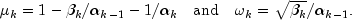Usually, the eigenvalue computation is needed for only a few of the iterations.

Example without preconditioning, Example with different preconditioners, Serialized Form
• ### Nested Class Summary

Nested Classes
Modifier and Type Class and Description
`static interface ` `ConjugateGradient.Function`
Public interface for the user supplied function to `ConjugateGradient`.
`static class ` `ConjugateGradient.NoConvergenceException`
The conjugate gradient method did not converge within the allowed maximum number of iterations.
`static class ` `ConjugateGradient.NotDefiniteAMatrixException`
The input matrix A is indefinite, that is the matrix is not positive or negative definite.
`static class ` `ConjugateGradient.NotDefiniteJacobiPreconditionerException`
The Jacobi preconditioner is not strictly positive or negative definite.
`static class ` `ConjugateGradient.NotDefinitePreconditionMatrixException`
The Precondition matrix is indefinite.
`static interface ` `ConjugateGradient.Preconditioner`
Public interface for the user supplied function to `ConjugateGradient` used for preconditioning.
`static class ` `ConjugateGradient.SingularPreconditionMatrixException`
The Precondition matrix is singular.
• ### Constructor Summary

Constructors
Constructor and Description
```ConjugateGradient(int n, ConjugateGradient.Function argF)```
• ### Method Summary

Methods
Modifier and Type Method and Description
`int` `getIterations()`
Returns the number of iterations needed by the conjugate gradient algorithm.
`double[]` `getJacobi()`
Returns the Jacobi preconditioning matrix.
`int` `getMaxIterations()`
Returns the maximum number of iterations allowed.
`double` `getRelativeError(double errorRelative)`
Returns the relative error used for stopping the algorithm.
`void` `setJacobi(double[] diagonal)`
Defines a Jacobi preconditioner as the preconditioning matrix, that is, M is the diagonal of `A`.
`void` `setMaxIterations(int maxIterations)`
Sets the maximum number of iterations allowed.
`void` `setRelativeError(double tolerance)`
Sets the relative error used for stopping the algorithm.
`double[]` `solve(double[] b)`
Solves a real symmetric positive or negative definite systemusing a conjugate gradient method with or without preconditioning.
• ### Methods inherited from class java.lang.Object

`clone, equals, finalize, getClass, hashCode, notify, notifyAll, toString, wait, wait, wait`
• ### Constructor Detail

```public ConjugateGradient(int n,
Parameters:
`n` - an `int` scalar value defining the order of the matrix.
`argF` - a `Function` that defines the user-supplied function which computes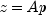. If `argF` implements `Preconditioner` then right preconditioning is performed using this user supplied function. Otherwise, no preconditioning is performed. Note that `argF` can be used to act upon the coefficients of matrix A stored in different storage modes.
• ### Method Detail

• #### getIterations

`public int getIterations()`
Returns the number of iterations needed by the conjugate gradient algorithm.
Returns:
an `int` value indicating the number of iterations needed.
• #### getJacobi

`public double[] getJacobi()`
Returns the Jacobi preconditioning matrix.
Returns:
a `double` vector `diagonal` containing the diagonal of the Jacobi preconditioner M, that is, `diagonal[i]`=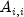, A the input matrix.
• #### getMaxIterations

`public int getMaxIterations()`
Returns the maximum number of iterations allowed.
Returns:
an `int` value specifying the maximum number of iterations allowed.
• #### getRelativeError

`public double getRelativeError(double errorRelative)`
Returns the relative error used for stopping the algorithm.
Returns:
a `double` containing the relative error.
• #### setJacobi

`public void setJacobi(double[] diagonal)`
Defines a Jacobi preconditioner as the preconditioning matrix, that is, M is the diagonal of `A`.
Parameters:
`diagonal` - a `double` vector containing the diagonal of A as the Jacobi preconditioner M, that is, `diagonal[i]`=.
Throws:
`IllegalArgumentException` - is thrown if the length of vector `diagonal` is not equal to the order `n` of input matrix A.
• #### setMaxIterations

`public void setMaxIterations(int maxIterations)`
Sets the maximum number of iterations allowed.
Parameters:
`maxIterations` - an `int` value specifying the maximum number of iterations allowed. By default, `maxIterations` =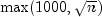.
Throws:
`IllegalArgumentException` - is thrown if `maxIterations` is less than or equal to 0.
• #### setRelativeError

`public void setRelativeError(double tolerance)`
Sets the relative error used for stopping the algorithm.
Parameters:
`tolerance` - a `double` specifying the relative error. By default, `tolerance` = 1.49e-08, the square root of the precision.
Throws:
`IllegalArgumentException` - is thrown if `tolerance` is less than 0.
• #### solve

```public double[] solve(double[] b)
SingularMatrixException,
Solves a real symmetric positive or negative definite systemusing a conjugate gradient method with or without preconditioning.
Parameters:
`b` - a `double` vector of length `n` containing the right-hand side.
Returns:
a `double` vector of length `n` containing the approximate solution to the linear system.
Throws:
`IllegalArgumentException` - is thrown if the length of `b` is not consistent with the order `n` of `A`.
`ConjugateGradient.SingularPreconditionMatrixException` - is thrown if the preconditioning matrix is singular.
`ConjugateGradient.NotDefinitePreconditionMatrixException` - is thrown if the preconditioning matrix is not definite.
`SingularMatrixException` - is thrown if input matrix A is singular.
`ConjugateGradient.NotDefiniteAMatrixException` - is thrown if matrix A is not definite.
`ConjugateGradient.NoConvergenceException` - is thrown if the algorithm is not convergent within `maxIterations` iterations.
`ConjugateGradient.NotDefiniteJacobiPreconditionerException` - is thrown if the Jacobi preconditioner is not definite.
JMSLTM Numerical Library 7.2.0